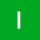Home Communities
IT Knowledge
Inspiration
Languages
EN

# Java - operators

0 points
Created by:illona
526

In this article, we would like to show you operators that are used to perform operations on variables and values in Java.

OperatorNameDescriptionExample
`+`additionadds together two valuesa + b
`-`subtractionsubtracts the second value from the first onea - b
`*`multiplicationmultiplies two valuesa * b
`/`divisiondivides the first value by the second onea / b
`%`modulusreturns the division remaindera % b
`++`incrementincreases the value of a variable by 1a++
`--`decrementdecreases the value of a variable by 1a--

## Practical examples

### 1. `+` operator

In this example, we use `+` operator to sum two values.

``````public class Example {

public static void main(String[] args) {
int result = 10 + 20;
System.out.println(result);  // 30
}
}``````

Output:

``30``

### 2. `-` operator

In this example, we use `-` operator to subtract two values.

``````public class Example {

public static void main(String[] args) {
int result = 20 - 10;
System.out.println(result);  // 10
}
}``````

Output:

``10``

### 3. `*` operator

In this example, we use `*` operator to multiply two values.

``````public class Example {

public static void main(String[] args) {
int result = 10 * 2;
System.out.println(result);  // 20
}
}``````

Output:

``20``

### 4. `/` operator

In this example, we use `/` operator to divide the first value by the second one.

``````public class Example {

public static void main(String[] args) {
int result = 20 / 2;
System.out.println(result);  // 10
}
}``````

Output:

``10``

### 5. `%` operator

In this example, we use `%` operator to get the division remainder.

``````public class Example {

public static void main(String[] args) {
int result = 10 % 3;
System.out.println(result);  // 1 (because 10/3=3 and 1 remains)
}
}``````

Output:

``1``

### 6. `++` operator

In this example, we use `++` operator to increment the `result` value.

``````public class Example {

public static void main(String[] args) {
int result = 10;
result++;
System.out.println(result);  // 11
}
}``````

Output:

### 7. `--` operator

In this example, we use `--` operator to decrement the `result` value.

``````public class Example {

public static void main(String[] args) {
int result = 10;
result--;
System.out.println(result);  // 9
}
}``````

Output:

``9``
Join to our subscribers to be up to date with content, news and offers.